# Free online courseStatistics by Leonard

4.85

(27)

## 31h55m of online video course

Quality content and selected for your learning.

## Exercises to train your knowledge

Many of the courses available have exercises to help with learning.

This course is free today and always will be, from start to finish you will pay nothing.

## StatisticsTeacher

##### Leonard
###### 6 Courses / 1743 students

This Channel is dedicated to quality mathematics education. It is absolutely FREE so Enjoy! Videos are organized in playlists and are course specific.

## Course content preview

There are no comments yet for this course.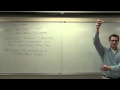Watch the 1st video of the course

## Course content0h17m

### Statistics Lecture 1.1: The Key Words and Definitions For Elementary Statistics

Statistics Lecture 1.1: The Key Words and Definitions For Elementary Statistics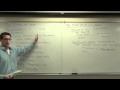0h31m

### Statistics Lecture 1.3: Exploring Categories of Data, Levels of Measurement

Statistics Lecture 1.3: Exploring Categories of Data, Levels of Measurement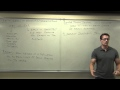0h31m

### Statistics Lecture 1.5: Sampling Techniques. How to Develop a Random Sample

Statistics Lecture 1.5: Sampling Techniques. How to Develop a Random Sample1h07m

### Statistics Lecture 2.2: Creating Frequency Distribution and Histograms

Statistics Lecture 2.2: Creating Frequency Distribution and Histograms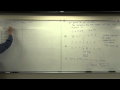1h11m

### Statistics Lecture 3.2: Finding the Center of a Data Set. Mean, Median, Mode

Statistics Lecture 3.2: Finding the Center of a Data Set. Mean, Median, Mode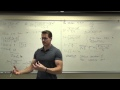1h56m

### Statistics Lecture 3.3: Finding the Standard Deviation of a Data Set

Statistics Lecture 3.3: Finding the Standard Deviation of a Data Set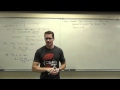1h31m

### Statistics Lecture 3.4: Finding Z-Score, Percentiles and Quartiles, and Comparing Standard Deviation

Statistics Lecture 3.4: Finding the Z-Score, Percentiles and Quartiles, and Comparing Standard Deviation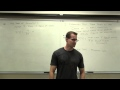1h42m

### Statistics Lecture 4.2: Introduction to Probability

Statistics Lecture 4.2: Introduction to Probability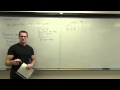1h09m

### Statistics Lecture 4.3: The Addition Rule for Probability

Statistics Lecture 4.3: The Addition Rule for Probability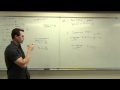1h06m

### Statistics Lecture 4.4: The Multiplication Rule for "And" Probabilities.

Statistics Lecture 4.4: The Multiplication Rule for "And" Probabilities.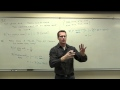0h32m

### Statistics Lecture 4.5: Probability of Complementary Events with "At Least One"

Statistics Lecture 4.5: Probability of Complementary Events with "At Least One"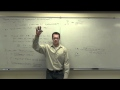1h37m

### Statistics Lecture 4.7: Fundamental Counting Rule, Permutations and Combinations

Statistics Lecture 4.7: Fundamental Counting Rule, Permutations and Combinations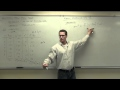1h12m

### Statistics Lecture 5.2: A Study of Probability Distributions, Mean, and Standard Deviation

Statistics Lecture 5.2: A Study of Probability Distributions, Mean, and Standard Deviation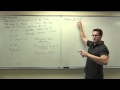1h32m

### Statistics Lecture 5.3: A Study of Binomial Probability Distributions

Statistics Lecture 5.3: A Study of Binomial Probability Distributions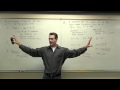0h42m

### Statistics Lecture 5.4: Finding Mean and Standard Deviation of a Binomial Probability Distribution

Statistics Lecture 5.4: Finding the Mean and Standard Deviation of a Binomial Probability Distribution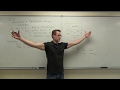2h11m

### Statistics Lecture 6.2: Introduction to the Normal Distribution and Continuous Random Variables

Statistics Lecture 6.2: Introduction to the Normal Distribution and Continuous Random Variables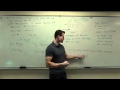0h56m

### Statistics Lecture 6.3: The Standard Normal Distribution. Using z-score, Standard Score

Statistics Lecture 6.3: Applications of the Standard Normal Distribution. Using z-score, Standard Score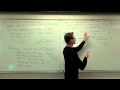0h50m

### Statistics Lecture 6.4: Sampling Distributions Statistics. Using Samples to Approx. Populations

Statistics Lecture 6.4: Sampling Distributions of Sample Statistics. Using Samples to Approx. Populations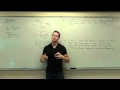1h31m

### Statistics Lecture 6.5: The Central Limit Theorem for Statistics. Using z-score, Standard Score

Statistics Lecture 6.5: The Central Limit Theorem for Statistics. Using z-score, Standard Score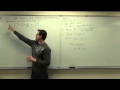2h24m

### Statistics Lecture 7.2: Finding Confidence Intervals for the Population Proportion

Statistics Lecture 7.2: Finding Confidence Intervals for the Population Proportion